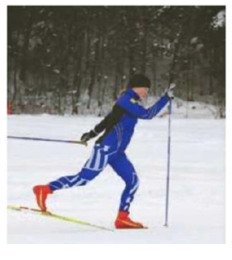Chapter 11, Problem 33P

Chapter
Section
Textbook Problem

A 75-kg cross-country skier glides over snow as in Figure P11.33. The coefficient of friction between skis and snow is 0.20. Assume ail the snow beneath her skis is at 0°C and that all the internal energy generated by friction is added to snow, which sticks to her skis until it melts. How far would she have 10 ski to melt 1.0 kg of snow?To determine
The distance that skier to go to melt 1.0 kg snow.

Explanation

Given Info: Mass of skier is 75 kg, temperature of snow is 0°C , mass of snow to melt is 1.0 kg and the coefficient of friction between skis and snow is 0.20.

All the work that is done against the friction is used to melt the snow.

Formula to calculate the work done against the friction is,

W=μkmskiergd                           (I)

• W is the work done against the friction,
• μk is the coefficient of kinetic friction,
• mskier is the mass of the skier,
• g is the acceleration due to gravity,
• d is the distance travelled,

Formula to calculate the heat required to melt the snow is,

Qmelt=msnowLf                           (II)

• Qmelt is the energy required to melt the ice to cold water,
• msnow is the mass of the ice,
• Lf is the latent heat of fusion of ice,

The work that is done against the friction is used to melt the snow.

W=Qmelt

Equate (I) and (II) and rewrite in terms of d.

μkmskiergd=msnowLfd=msnowLfμkmskierg

Substitute 1

Still sussing out bartleby?

Check out a sample textbook solution.

See a sample solution

The Solution to Your Study Problems

Bartleby provides explanations to thousands of textbook problems written by our experts, many with advanced degrees!

Get Started

Find more solutions based on key concepts